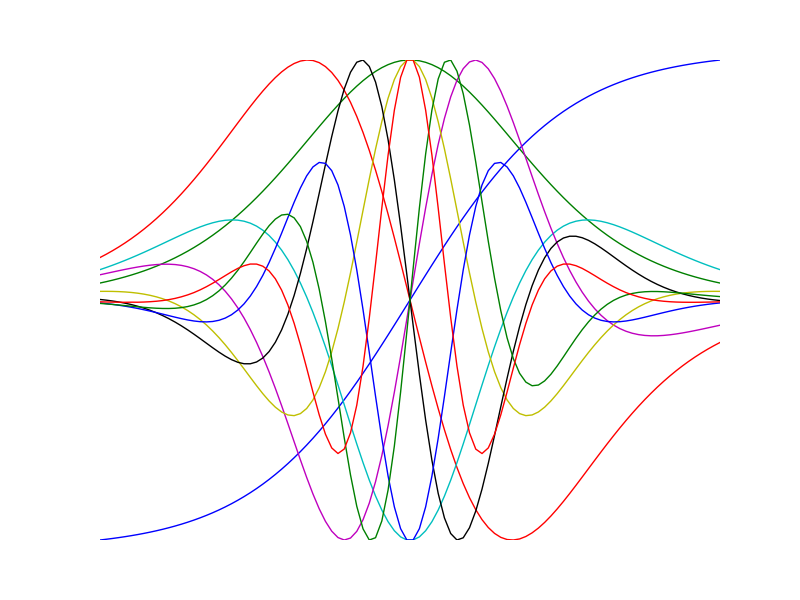Solves automatic numerical differentiation problems in one or more variables.

## numdifftools

The numdifftools library is a suite of tools written in _Python to solve automatic numerical differentiation problems in one or more variables. Finite differences are used in an adaptive manner, coupled with a Richardson extrapolation methodology to provide a maximally accurate result. The user can configure many options like; changing the order of the method or the extrapolation, even allowing the user to specify whether complex-step, central, forward or backward differences are used.

The methods provided are:

• Derivative: Compute the derivatives of order 1 through 10 on any scalar function.

• directionaldiff: Compute directional derivative of a function of n variables

• Gradient: Compute the gradient vector of a scalar function of one or more variables.

• Jacobian: Compute the Jacobian matrix of a vector valued function of one or more variables.

• Hessian: Compute the Hessian matrix of all 2nd partial derivatives of a scalar function of one or more variables.

• Hessdiag: Compute only the diagonal elements of the Hessian matrix

All of these methods also produce error estimates on the result.

Numdifftools also provide an easy to use interface to derivatives calculated with in _AlgoPy. Algopy stands for Algorithmic Differentiation in Python. The purpose of AlgoPy is the evaluation of higher-order derivatives in the forward and reverse mode of Algorithmic Differentiation (AD) of functions that are implemented as Python programs.

### Getting Started

Visualize high order derivatives of the tanh function

>>> import numpy as np
>>> import numdifftools as nd
>>> import matplotlib.pyplot as plt
>>> x = np.linspace(-2, 2, 100)
>>> for i in range(10):
...    df = nd.Derivative(np.tanh, n=i)
...    y = df(x)
...    h = plt.plot(x, y/np.abs(y).max())

>>> plt.show() # doctest: +SKIPCompute 1’st and 2’nd derivative of exp(x), at x == 1:

>>> fd = nd.Derivative(np.exp)        # 1'st derivative
>>> fdd = nd.Derivative(np.exp, n=2)  # 2'nd derivative
>>> np.allclose(fd(1), 2.7182818284590424)
True
>>> np.allclose(fdd(1), 2.7182818284590424)
True

Nonlinear least squares:

>>> xdata = np.reshape(np.arange(0,1,0.1),(-1,1))
>>> ydata = 1+2*np.exp(0.75*xdata)
>>> fun = lambda c: (c+c*np.exp(c*xdata) - ydata)**2
>>> Jfun = nd.Jacobian(fun)
>>> np.allclose(np.abs(Jfun([1,2,0.75])), 0) # should be numerically zero
True

>>> fun = lambda x: np.sum(x**2)
>>> np.allclose(dfun([1,2,3]), [ 2.,  4.,  6.])
True

Compute the same with the easy to use interface to AlgoPy:

>>> import numdifftools.nd_algopy as nda
>>> import numpy as np
>>> fd = nda.Derivative(np.exp)        # 1'st derivative
>>> fdd = nda.Derivative(np.exp, n=2)  # 2'nd derivative
>>> np.allclose(fd(1), 2.7182818284590424)
True
>>> np.allclose(fdd(1), 2.7182818284590424)
True

Nonlinear least squares:

>>> xdata = np.reshape(np.arange(0,1,0.1),(-1,1))
>>> ydata = 1+2*np.exp(0.75*xdata)
>>> fun = lambda c: (c+c*np.exp(c*xdata) - ydata)**2
>>> Jfun = nda.Jacobian(fun, method='reverse')
>>> np.allclose(np.abs(Jfun([1,2,0.75])), 0) # should be numerically zero
True

>>> fun = lambda x: np.sum(x**2)
>>> np.allclose(dfun([1,2,3]), [ 2.,  4.,  6.])
True

scipy.misc.derivative

### Documentation and code

Numdifftools works on Python 2.7+ and Python 3.0+.

Bleeding edge: https://github.com/pbrod/numdifftools.

### Installation

If you have pip installed, then simply type:

\$ pip install numdifftools

to get the lastest stable version. Using pip also has the advantage that all requirements are automatically installed.

### Unit tests

To test if the toolbox is working paste the following in an interactive python session:

import numdifftools as nd
nd.test('--doctest-modules', '--disable-warnings')

### Acknowledgement

The numdifftools package for Python was written by Per A. Brodtkorb based on the adaptive numerical differentiation toolbox written in Matlab by John D’Errico [DErrico06].

Later the package was extended with some of the functionality found in the statsmodels.tools.numdiff module written by Josef Perktold [JPerktold14] which is based on [Rid09]. The implementation of bicomplex numbers is based on the matlab implementation described in the project report of [Ver14] which is based on [GLD12]. For completeness the [For98] method for computing the weights and points in general finite difference formulas as well as the [For81] method for cumputing the taylor coefficients of complex analytic function using FFT, was added.

## Project details

This version0.9.410.9.400.9.390.9.380.9.200.9.170.9.160.9.150.9.140.9.130.9.120.9.110.9.100.9.20.7.70.7.30.6.00.5.00.4.00.3.50.3.40.3.30.3.10.2.10.2

Uploaded source
Uploaded py2 py3